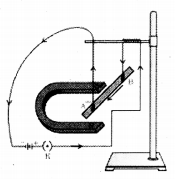# (a) Describe with diagram an experiment to show that a force is exerted on a current carrying conductor

(a) Describe with diagram an experiment to show that a force is exerted on a current carrying conductor when placed perpendicular in a magnetic field.
(b) State the rule to find the direction of the force exerted on a current carrying conductor in a magnetic field.

(a)Using a horse shoe magnet, a current carrying conductor is placed in such a way that it lies perpendicular to the magnetic field. When the key K is closed, the conductor AB moves such that it is perpendicular to both magnetic field as well as the direction of current.

(b) A current carrying conductor, when placed perpendicular to magnetic field, experiences force. The direction of this force is given by Fleming’s left hand rule. On stretching the forefinger, middle finger, and thumb of the left hand perpendicular to one another, the forefinger points in the direction of magnetic field, the middle finger points in the direction of current, and the direction in which the thumb points gives the direction of force.